Error message - WoWInterface
07-10-16, 01:28 PM   #1
stones
A Black Drake
Join Date: Jan 2013
Posts: 85
Error message

Iv been getting this error message every time I log into one of my toons. I sent this zygor and they said it isn't there addone. So I un checked all my addons and re checked them one at a time and no error with all of them I ret the error can someone help please?

Message: Interface\AddOns\ZygorGuidesViewer\Pointer.lua:4093: attempt to perform arithmetic on field 'width' (a nil value)
Time: 07/10/16 12:03:54
Count: 9
[C]: ?

Locals: t = <table> {
4 = 2.64375
9 = 2.7249998779297
11 = 2.8729166641235
13 = 10
14 = 10
16 = 1.7385416259766
17 = 1.5354166259766
19 = 1.83125
20 = 2.2593749389648
21 = 2.0999998779297
22 = 2.1499999542236
23 = 2.015625
24 = 2.4312499389648
26 = 1.925
27 = 2.4489582519531
28 = 1.1156249237061
29 = 1.576041595459
30 = 1.7354166259766
32 = 1.2499999694824
775 = 0.5506404876709
35 = 1.3791665649414
37 = 2.0499999389648
39 = 1.7499999084473
903 = 0.1095
935 = 0.5506404876709
776 = 0.5506404876709
808 = 0.5506404876709
485 = 8.875699195
501 = 1.4874999389648
522 = 0.5506404876709
809 = 3.1291665039063
873 = 0.896875
905 = 0.1
937 = 0.5506404876709
969 = 0.5506404876709
523 = 0.5506404876709
810 = 2.8718745117188
874 = 0.5506404876709
906 = 0.5506404876709
938 = 0.5506404876709
970 = 0.5506404876709
502 = 1.581246925
524 = 0.5506404876709
811 = 1.2666669921875
875 = 0.5506404876709
939 = 0.5506404876709
525 = 0.5506404876709
684 = 1.5729166259766
989 = 0.5506404876709
876 = 0.5506404876709
281 = 3.0749999389648
685 = 0.44479162597656
717 = 1.2562456
749 = 0.5506404876709
526 = 0.5506404876709
723 = 0.5506404876709
609 = 0.376041655
877 = 0.5506404876709
504 = 0.415008215
941 = 3.0572899780273
718 = 0.5506404876709
261 = 2.0291666259766
782 = 0.5506404876709
807 = 1.9625004882813
871 = 0.5506404876709
878 = 0.5506404876709
41 = 2.9374998779297
687 = 0.5506404876709
488 = 2.8041665649414
751 = 0.1
528 = 0.5506404876709
141 = 2.625
341 = 0.39531253051758
181 = 2.7572917480469
911 = 0.5506404876709
462 = 2.462499675
720 = 3.0968748779297
752 = 0.5506404876709
61 = 2.1999998474121
816 = 0.5506404876709
603 = 0.5506404876709
880 = 0.5506404876709
912 = 0.5506404876709
473 = 2.75
721 = 0.5506404876709
1008 = 0.5506404876709
530 = 0.5506404876709
951 = 1.2
486 = 2.8822915039063
747 = 0.485418135
779 = 0.5506404876709
690 = 0.5506404876709
362 = 0.52187496948242
43 = 2.883333190918
531 = 0.581249805
945 = 2.6249999389648
381 = 0.76979168701172
780 = 0.5506404876709
914 = 0.5506404876709
946 = 2.9812480392456
978 = 1.561455078125
897 = 0.5506404876709
532 = 0.5506404876709
201 = 1.8499999084473
851 = 0.5506404876709
940 = 0.5506404876709
781 = 0.5506404876709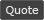07-13-16, 11:44 AM   #2
JWMcQuire
A Flamescale Wyrmkin
Join Date: Jun 2013
Posts: 107
Could you please post a list of your addons and which version of Carbonite you are using?

Also, you may want to disable "TomTom Emulation" if you have TomTom installed to see if that fixes your issue.

Edit:
Zygor, based on its description on the website is a guide much like WoWPro. The difference is that it has its own built-in quest tracking/waypoint code. This means that it doesn't require Carbonite or TomTom to work as WoWPro does, and many people I know who use WoWPro prefer TomTom even though WoWPro now patches what it calls "Carbonite's fake TomTom".

So since Zygor has its own tracking system, it may be possible that it won't play nice with Carbonite and making them play nice together is not as high a priority as getting Carbonite ready for Legion.

That said, if login is the only time the error occurs and they work well together after that, you may want to ignore the error and play on as usual.
__________________
I am not an addon author. I contribute when I can, answer questions when I am able, and post issues or ask questions now and then.

Last edited by JWMcQuire : 07-13-16 at 12:50 PM. Reason: Added note about Zygor07-13-16, 01:14 PM #3 myrroddin A Pyroguard Emberseer Join Date: Oct 2008 Posts: 1,109 Definitely an error with Zygor. According to their forums, they have had access to the Legion beta for some time, and are working on a compatible version, which will launch at or close to Legion's launch. The Legion version of Zygor will be available for pre-order soon(tm). As for your error, the first four lines clearly mention ZygorGuidesViewer. Lua Code: `Message: Interface\AddOns\ZygorGuidesViewer\Pointer.lua:4093: attempt to perform arithmetic on field 'width' (a nil value)`WoWInterface » Error message

 Thread Tools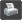Show Printable Version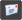Email this Page Display Modes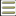Linear Mode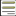Switch to Hybrid Mode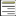Switch to Threaded Mode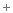Posting Rules You may not post new threads You may not post replies You may not post attachments You may not edit your posts vB code is On Smilies are On [IMG] code is On HTML code is Off